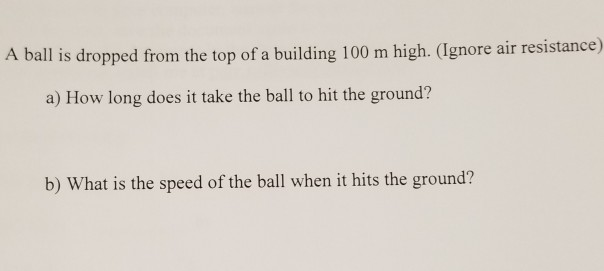Question
A 3.79 kg ball is dropped from the roof of
a building 163.8 m high. While the ball is
falling to Earth, a horizontal wind exerts a constant force of 11.5 N on the ball. How long does it take to hit the ground?
The acceleration of gravity is 9.81 m/s2 .
Answer in units of s.

The dynamics of the horizontal problem have nothing to do with the falling problem in the vertical direction.
The mass of the ball is also irrelevant.
This is simply something falling 163.8 meters, period.

163.8 = (1/2)(9.81) t^2
solve for t

Perhaps later there is a part two where you will be asked how far from a point directly under the drop the ball lands, but that has not been asked yet.

#### Earn Coins

Coins can be redeemed for fabulous gifts.

Similar Homework Help Questions
• ### A 3.79 kg ball is dropped from the roof of a building 163.8 m high

A 3.79 kg ball is dropped from the roof of a building 163.8 m high. While the ball is falling to Earth, a horizontal wind exerts a constant force of 11.5 N on the ball. It takes 5.77 second to hit the ground. How far from the building does the ball hit the ground? Answer in units of m.

• ### A 3.61 kg ball is dropped from the roof of a building 152.5 m high

A 3.61 kg ball is dropped from the roof of a building 152.5 m high. While the ball is falling to Earth, a horizontal wind exerts a constant force of 11.6 N on the ball. How long does it take to hit the ground? The acceleration of gravity is 9.81 m/s^2

• ### A 3.60 kg ball is dropped from the roof of a building 184.8 m high

A 3.60 kg ball is dropped from the roof of a building 184.8 m high. While the ball is falling to earth, a horizontal wind exerts a constant force of 12.1 N on the ball. How long does it take to hit the ground? (acceleration of gravity = 9.81 m/s^2)

• ### A 3.60 kg ball is dropped from the roof of a building 184.8 m high

A 3.60 kg ball is dropped from the roof of a building 184.8 m high. While the ball is falling to earth, a horizontal wind exerts a constant force of 12.1 N on the ball. (acceleration of gravity = 9.81 m/s^2) How far from the building does the ball hit the ground? What is the speed when it hits the ground?

• ### A 3.00 kg ball is dropped from the roof of a building 176.4 m high

A 3.00 kg ball is dropped from the roof of a building 176.4 m high. While the ball is falling to Earth, a horizontal wind exerts a constant force of 12.0 N on it. a.)How far from the building does the ball hit the ground? b.)How long does it take to hit the ground? c.)What is its speed when it hits the ground?

• ### A ball is thrown straight up from the ground with an initial velocity of 30.4 m/s; at the same instant, a ball is dropped from the roof of a building 21.1 m high

A ball is thrown straight up from the ground with an initial velocity of 30.4 m/s; at the same instant, a ball is dropped from the roof of a building 21.1 m high. After how long will the balls be at the same height? The acceleration of gravity is 10 m/s2. Answer in units of s

• ### A ball is dropped from the top of a building 100 m high. (Ignore air resistance)...A ball is dropped from the top of a building 100 m high. (Ignore air resistance) a) How long does it take the ball to hit the ground? b) What is the speed of the ball when it hits the ground?

• ### A ball dropped from the roof of a building take 4s to reach the street

A ball dropped from the roof of a building take 4s to reach the street. How high is the building?

• ### a ball with mass 0.15 kg is thrown upward with initial velocity 20 m/sec from the roof of a building 30 m high

a ball with mass 0.15 kg is thrown upward with initial velocity 20 m/sec from the roof of a building 30 m high. there is a force due to air resistance of |v|/30, where velocity v is measured in m/sec.a. find the maximum height above the ground the ball reaches.b. find the time the ball hits the ground.you cannot use the kinematic equations.

• ### A ball is thrown horizontally from the top of a building 55.4 m high

A ball is thrown horizontally from the top of a building 55.4 m high. The ball strikes the ground at a point 65 m from the base of the building. The acceleration of gravity is 9.8 m/s2 . Find the time the ball is in motion in seconds.